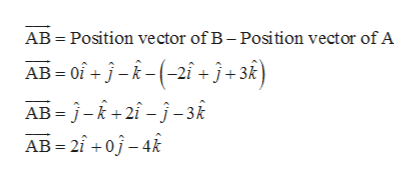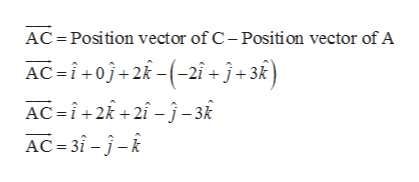# Find the angle ∠BAC if A = (−2, 1, 3), B = (0, 1, −1) and C = (1, 0, 2)

Question
94 views

Find the angle ∠BAC if A = (−2, 1, 3), B = (0, 1, −1) and C = (1, 0, 2)

check_circle

Step 1

Consider the given vertices.

A = (-2, 1, 3), B = (0, 1, -1), C = (1, 0, 2)

Step 2

Now, find the vectors of the given vertices.

Vector AB ishelp_outlineImage TranscriptioncloseAB Position vector of B- Position vector of A AB of+jR2i+j+3k) AB j-k+2i-j-3k AB 20-4 fullscreen
Step 3

Similarly, vect...help_outlineImage TranscriptioncloseAC Position vector of C- Position vector of A AC i+0j+2(-2i + j+ 3k ) AC i 2 -3 AC 3i--k fullscreen

### Want to see the full answer?

See Solution

#### Want to see this answer and more?

Solutions are written by subject experts who are available 24/7. Questions are typically answered within 1 hour.*

See Solution
*Response times may vary by subject and question.
Tagged in

### Calculus